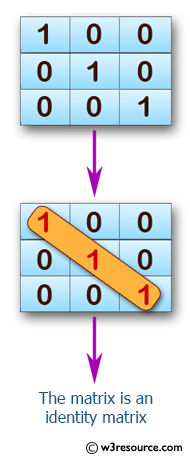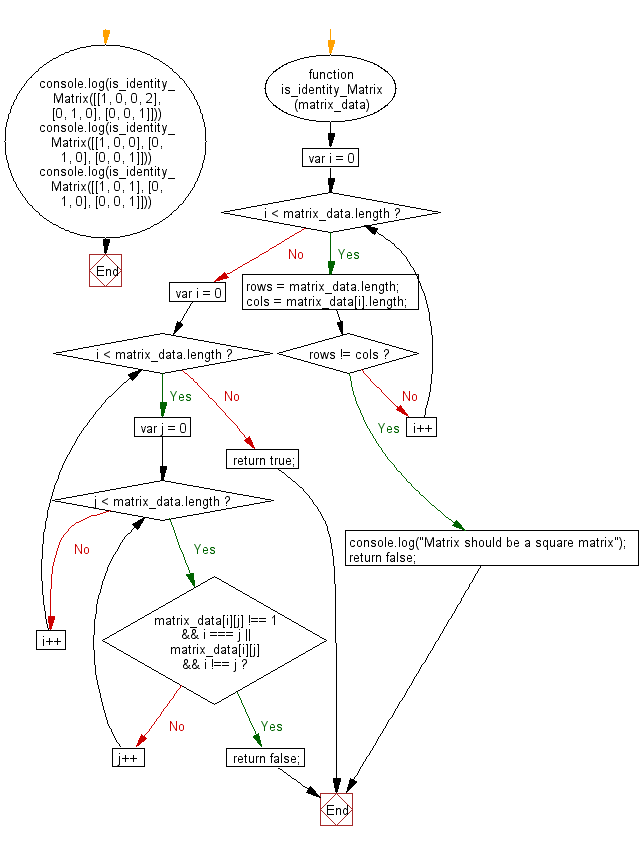# JavaScript: Check whether a given matrix is an identity matrix

## JavaScript Basic: Exercise-117 with Solution

Write a JavaScript program to check whether a given matrix is an identity matrix.

Note: In linear algebra, the identity matrix, or sometimes ambiguously called a unit matrix, of size n is the n × n square matrix with ones on the main diagonal and zeros elsewhere.
[[1, 0, 0], [0, 1, 0], [0, 0, 1]] -> true
[[1, 0, 0], [0, 1, 0], [1, 0, 1]] -> false

Pictorial Presentation:Sample Solution:

HTML Code:

``````<!DOCTYPE html>
<html>
<meta charset="utf-8">
<meta name="viewport" content="width=device-width">
<title> Check if a given matrix is an identity matrix</title>
<body>

</body>
</html>
```
```

JavaScript Code:

``````function is_identity_Matrix(matrix_data) {
//Checks whether given matrix is a square matrix or not
for (var i = 0; i < matrix_data.length; i++)
{
rows = matrix_data.length;
cols = matrix_data[i].length;
if(rows != cols)
{
console.log("Matrix should be a square matrix");
return false;
}
}
for (var i = 0; i < matrix_data.length; i++)
{
for (var j = 0; j < matrix_data.length; j++)
{
if (matrix_data[i][j] !== 1 && i === j || matrix_data[i][j] && i !== j)
{
return false;
}
}
}
return true;
}
console.log(is_identity_Matrix([[1, 0, 0, 2], [0, 1, 0], [0, 0, 1]]))
console.log(is_identity_Matrix([[1, 0, 0],  [0, 1, 0],  [0, 0, 1]]))
console.log(is_identity_Matrix([[1, 0, 1],  [0, 1, 0],  [0, 0, 1]]))
``````

Sample Output:

```Matrix should be a square matrix
false
true
false
```

Flowchart:ES6 Version:

``````function is_identity_Matrix(matrix_data) {
for (let i = 0; i < matrix_data.length; i++)
{
for (let j = 0; j < matrix_data.length; j++)
{
if (matrix_data[i][j] !== 1 && i === j || matrix_data[i][j] && i !== j)
{
return false;
}
}
}
return true;
}

console.log(is_identity_Matrix([[1, 0, 0],  [0, 1, 0],  [0, 0, 1]]))
console.log(is_identity_Matrix([[1, 0, 1],  [0, 1, 0],  [0, 0, 1]]))
``````

Live Demo:

See the Pen javascript-basic-exercise-117 by w3resource (@w3resource) on CodePen.

Improve this sample solution and post your code through Disqus

What is the difficulty level of this exercise?

Test your Programming skills with w3resource's quiz.

﻿

## JavaScript: Tips of the Day

Returns the sum of the powers of all the numbers from start to end (both inclusive)

Example:

```const sumPower = (end, power = 2, start = 1) =>
Array(end + 1 - start)
.fill(0)
.map((x, i) => (i + start) ** power)
.reduce((a, b) => a + b, 0);
console.log(sumPower(10)); // 385
console.log(sumPower(10, 3)); // 3025
console.log(sumPower(10, 3, 5)); // 2925
```

Output:

```385
3025
2925
```